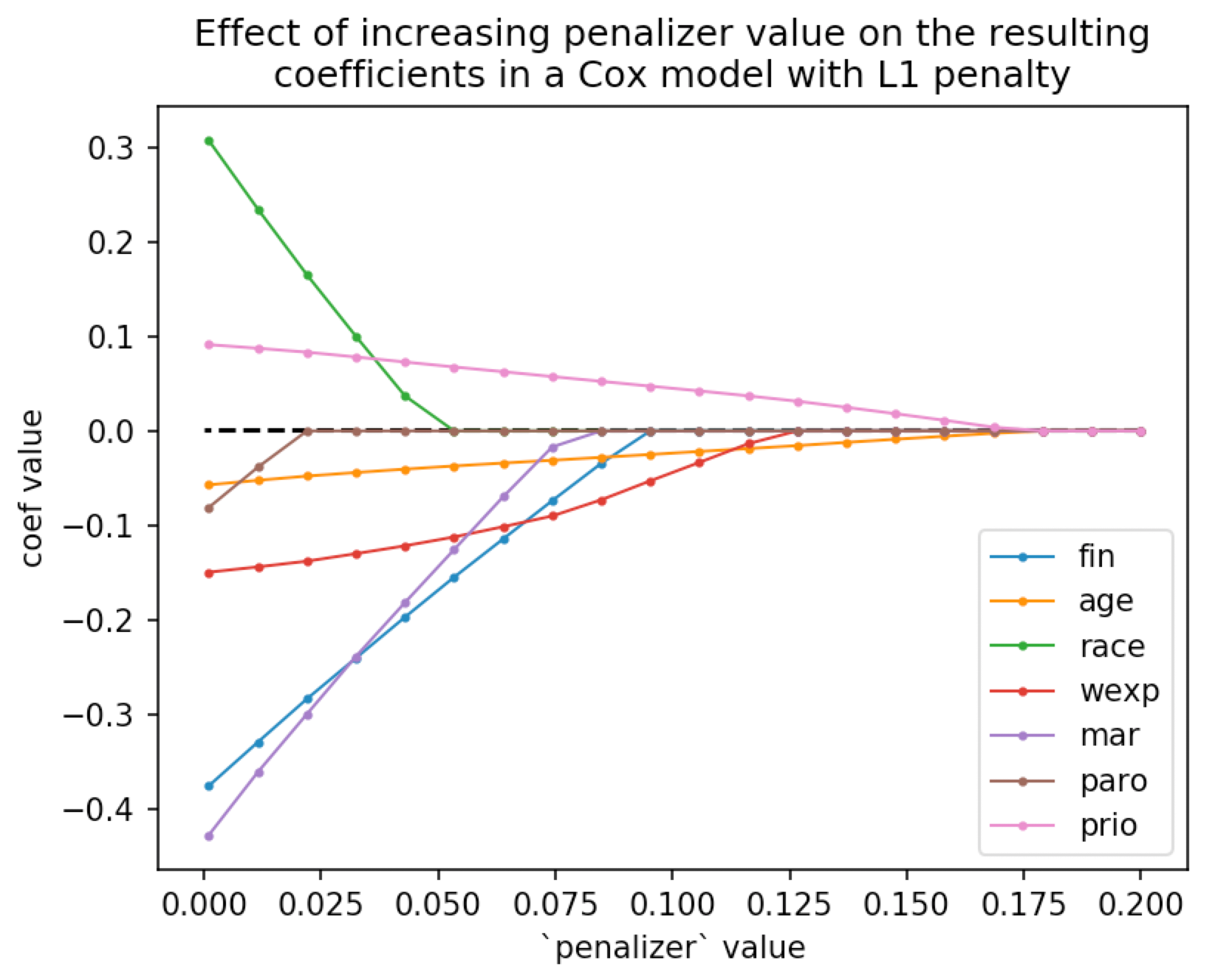# L₁ Penalty in Cox Regression

Posted by Cameron Davidson-Pilon on

In the 00's, L1 penalties were all the rage in statistics and machine learning. Since they induced sparsity in fitted parameters, they were used as a variable selection method. Today, with some advanced models having tens of billions of parameters, sparsity isn't as useful, and the L1 penalty has dropped out of fashion.

However, most teams aren't using billion parameter models, and smart data scientists work with simple models initially. Below is how we implemented an L1 penalty in the Cox regression model.

## Smoothing the absolute value

The log-likelihood we wish to maximize looks like:

$$ll^*(\theta, x) = \sum_i^N ll(\theta, x_i) - \lambda||\theta||_1$$

where $$||\cdot ||_1$$ is the sum of absolute values of the parameter vector $$\theta$$. With L2 penalties, the penalty term is differentiable, and we can easily find the gradient w.r.t. the parameter vector, which enables us to use iterative solvers to solve this optimization problem. However, when our penalty is L1, we don't have a smooth derivative.

We solve this by replacing $$||\cdot ||_1$$ with a smooth version:

$$\text{softabs}(\theta, a) = \frac{1}{a} \log(1 + \exp(-a\theta)) + \frac{1}{a}\log(1 + \exp(a\theta))$$

As $$a→\inf$$, this converges to the absolute value of $$\theta$$. This smooth absolute value is differentiable everywhere too. With that in mind, we can use our iterative solvers again, and increase $$a$$ each iteration. I don't think there is any simple solution to choose $$a$$, but I've found that increasing it exponentially works well.

## Elastic net penalty

We can combine this L1 penalty with an L2 penalty to get the elastic-net penalty, introduce a new parameter $$\rho$$ to control weighting between the two:

$$ll^*(\theta, x, a) = \sum_i^N ll(\theta, x_i) - \lambda(\rho||\theta||_2^2 + (1-\rho) \text{softabs}(\theta, a))$$

And remember: cool kids don't take derivatives by hand! Why waste time and make mistakes trying to compute the first and second derivative of this penalty - let autograd do it!

from autograd import elementwise_grad
from autograd import numpy as anp

def soft_abs(x, a):
return 1 / a * (anp.logaddexp(0, -a * x) + anp.logaddexp(0, a * x))

def penalizer(beta, a, lambda_, l1_ratio):    return lambda_ * (l1_ratio * soft_abs(beta, a).sum() + (1 - l1_ratio) * (beta ** 2).sum())

i = 0
while converging:
i += 1

h, g, ll = get_gradients(X, beta)

ll -= penalizer(beta, 1.3 ** i, 0.1, 0.5)
g -= d_penalizer(beta, 1.3 ** i, 0.1, 0.5)
h[np.diag_indices(d)] -= dd_penalizer(beta, 1.3 ** i, 0.1, 0.5)

# update beta and converging logic! Not shown here.


## Example in Cox model

In lifelines 0.24+, we introduced the L1 penalty for Cox models. We can visualize the sparsity effect of the L1 penalty as we increase the penalizer term:

from lifelines import CoxPHFitter

results = {}
for p in np.linspace(0.001, 0.2, 40):
cph = CoxPHFitter(l1_ratio=1., penalizer=p).fit(rossi, "week", "arrest")
results[p] = cph.params_

pd.DataFrame(results).T.plot()